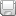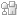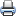# WDM Network DesignSaveDiggDel.icio.usPrint

### Chapter Description

This sample chapter explores some of the design constraints involved in the WDM network design.

## Design Using Chromatic Dispersion Compensation

In a chromatic dispersion-limited system in which the total accumulated dispersion for a traveling pulse is greater than the maximum allowable dispersion, the system cannot function because of tremendous ISI or just pure pulse spread. Therefore, we need to place dispersion compensation units (DCUs) at different positions in a network. Chapter 3 discussed some of the dispersion-compensating schemes, such as dispersion shifted fibers and FBGs, which are the most common. When we are designing a high bit rate WDM link (where dispersion can be considered a major design impairment), we should use dispersion maps to effectively design a system.

Dispersion maps are two-dimensional maps that plot the accumulated dispersion versus the length of transmission. They are particularly useful maps that help designers tell where to place dispersion compensators in a network. Accumulated dispersion is calculated by multiplying the fiber and the laser dispersion specifications for a given bit rate with respect to the length of the fiber. For example, an SMF fiber's typical value of dispersion is 16 ps/nm-km, which means that for every traversed kilometer of SMF fiber, a pulse at 10 Gbps (100 ps pulse width) spreads for about 16 ps from its mean. Ensure that the accumulated pulse spread across 'x' km is less than the maximum dispersion limit (which might be 1600 ps/km-nm for a 10 Gbps signal).

From this discussion, it is obvious that the signal can travel 16x = 1600 km (if x = 100) of SMF fiber at a 10 Gbps bit rate. It is important to note that as the signal traverses a greater distance, the accumulated dispersion also increases. For a given bit rate and at a given operating wavelength (or operating band), the maximum allowable accumulated dispersion is given by a standard specification. At no point in the dispersion map should the value of the curve go higher than the dispersion tolerance limit. Note that the dispersion parameters depend on many factors. The main factors are the bit rate (which gives the pulse width), the length of the fiber, the basic dispersion parameter, and the spectral width of the laser, which qualitatively provides the amount of dispersion induced (GVD). An interesting speculation is that of the variation of power penalty for dispersion-limited systems as a function of the dispersion parameter D, which is derived from the specification of the basic fiber. D can be considered a balancing component between the bit rate, the length of the fiber, and the width of the spectral source that emits the pulse. Refer to Figure 4-8.Figure 4-8 Variation of Dispersion Parameter D with Power Penalty

Two techniques—precompensation and postcompensation—can compensate dispersion using any of these methods. As the name implies, precompensation means compensating for dispersion before the signal is induced in the system. This is a technique of compressing the pulse in advance with DCUs; it takes care of the accumulated dispersion in advance. In contrast, postcompensation uses compensating equipment that is placed at the end of a fiber. In precompensation, we can place the DCU after the postline amplifier. Such units have loops of fiber with dispersion profile opposite to that of the transmission fiber. For example, a transmission fiber would have dispersion parameter of 16 ps/nm-km. A DCU could hypothetically be made to have a dispersion profile of – ~ 50 ps/nm-km. The signal passes through such fiber spools (DCU) and the pulse is precompensated. Conversely, with postcompensation techniques, the DCU modules are placed before the preline amplifier, as shown in Figure 4-9. Table 4-3 shows the dispersion parameter D for different kinds of fiber at 1550 nm.Figure 4-9 DCU Positioning in a Multistage System (Above) and Dispersion Map for the Same System (Below). Note the fall in dispersion due to the DCU placement and also note that accumulated dispersion never exceeds threshold (dotted line). Preceding technique is for precompensation.

#### Table 4-3 Dispersion Parameter D

 Fiber Type Normal Dispersion at 1550 nm Measured in ps/nm-km Single mode fiber (SMF) 17 E-Large Effective area fiber (ELEAF) 4 TrueWave RS (TW-RS) 4.2 Dispersion shifted fiber (DSF) -0.33

A serious loss (attenuation) occurs when DCUs are added. This is due to a coupling difference between transmission fiber and the DCU. Moreover, different dispersion profiles result in a phase mismatch, which prevents FWM from happening. This is one advantage of DCU in limiting the effects of nonlinearity. Refer to Figures 4-9 and 4-10.Figure 4-10 Dispersion Maps for Postcompensation Scheme

8. OSNR and Dispersion-Based Design | Next Section Previous Section